Gear ratio

Gear ratio is the ratio between the number of teeth on two gears that are meshed together, or two sprockets connected with a common roller chain, or the circumferences of two pulleys connected with a drive belt.

General description

In the picture to the right, the smaller gear (known as the pinion) has 13 teeth, while the second, larger gear (known as the idler gear) has 21 teeth. The gear ratio is therefore 13/21 or 1/1.62 (also written as 1:1.62).

This means that for every one revolution of the pinion, the gear has made 1/1.62, or 0.62, revolutions. In practical terms, the gear turns more slowly.

Suppose the largest gear in the picture has 42 teeth, the gear ratio between the second and third gear is thus 21/42 = 1/2, and for every revolution of the smallest gear the largest gear has only turned 0.62/2 = 0.31 revolution, a total reduction of about 1:3.23.

Since the intermediate (idler) gear contacts directly both the smaller and the larger gear it can be removed from the calculation, also giving a ratio of 42/13 = 3.23.

Since the number of teeth is also proportional to the circumference of the gear wheel (the bigger the wheel the more teeth it has) the gear ratio can also be expressed as the relationship between the circumferences of both wheels (where d is the diameter of the smaller wheel and D is the diameter of the larger wheel):$gr = \frac{\pi d}{\pi D} = \frac{d}{D}$

Since the diameter is equal to twice the radius;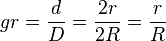$gr = \frac{d}{D} = \frac{2r}{2R} = \frac{r}{R}$

as well.$v_d = v_D \rightarrow \omega_d r = \omega_D R \rightarrow \frac{r}{R} = \frac{\omega_D}{\omega_d}$

and so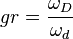$gr = \frac{\omega_D}{\omega_d}$

In other words, the gear ratio is proportional to ratio of the gear diameters and inversely proportional to the ratio of gear speeds.

Belts can have teeth in them also and be coupled to gear-like pulleys. Special gears called sprockets can be coupled together with chains, as on bicycles and some motorcycles. Again, exact accounting of teeth and revolutions can be applied with these machines.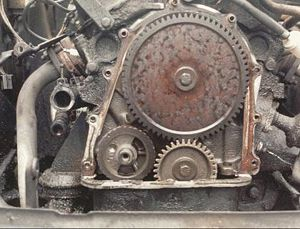Valve timing gears on a Ford Taunus V4 engine — the small gear is on the crankshaft, the larger gear is on the camshaft. The crankshaft gear has 34 teeth, the camshaft gear has 68 teeth and runs at half the crankshaft RPM.
(The small gear in the lower left is on the balance shaft.)

A belt with teeth, called the timing belt, is used in some internal combustion engines to exactly synchronize the movement of the camshaft with that of the crankshaft, so that the valves open and close at the top of each cylinder at exactly the right time relative to the movement of each cylinder. From the time the car is driven off the lot, to the time the belt needs replacing thousands of kilometers later, it synchronizes the two shafts exactly. A chain, called a timing chain, is used on some automobiles for this purpose, while in others, the camshaft and crankshaft are coupled directly together through meshed gears. But whichever form of drive is employed, on four-stroke engines the crankshaft/camshaft gear ratio is always 2:1, which means that for every two revolutions of the crankshaft the camshaft will rotate through one revolution.

Automobile drivetrains generally have two or more areas where gearing is used: one in the transmission, which contains a number of different sets of gearing that can be changed to allow a wide range of vehicle speeds, and another at the differential, which contains one additional set of gearing that provides further mechanical advantage at the wheels. These components might be separate and connected by a driveshaft, or they might be combined into one unit called a transaxle.

A 2004 Chevrolet Corvette C5 Z06 with a six-speed manual transmission has the following gear ratios in the transmission:

Gear Ratio
1st gear 2.97:1
2nd gear 2.07:1
3rd gear 1.43:1
4th gear 1.00:1
5th gear 0.84:1
6th gear 0.56:1
reverse 3.28:1

In 1st gear, the engine makes 2.97 revolutions for every revolution of the transmission’s output. In 4th gear, the gear ratio of 1:1 means that the engine and the transmission’s output are moving at the same speed. 5th and 6th gears are known as overdrive gears, in which the output of the transmission is revolving faster than the engine.

The above Corvette has a differential ratio of 3.42:1. This means that for every 3.42 revolutions of the transmission’s output, the wheels make one revolution. The differential ratio multiplies with the transmission ratio, so in 1st gear, the engine makes 10.16 revolutions for every revolution of the wheels.

The car’s tires can almost be thought of as a third type of gearing. The example Corvette Z06 is equipped with 233/45-14 tires, which have a circumference of 82.1 inches. This means that for every complete revolution of the wheel, the car travels 82.1 inches. If the Corvette had larger tires, it would travel farther with each revolution of the wheel, which would be like a higher gear. If the car had smaller tires, it would be like a lower gear.

With the gear ratios of the transmission and differential, and the size of the tires, it becomes possible to calculate the speed of the car for a particular gear at a particular engine RPM.

For example, it is possible to determine the distance the car will travel for one revolution of the engine by dividing the circumference of the tire by the combined gear ratio of the transmission and differential.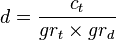$d = \frac{c_t}{gr_t \times gr_d}$

It is possible to determine a car’s speed from the engine speed by multiplying the circumference of the tire by the engine speed and dividing by the combined gear ratio.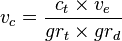$v_c = \frac{c_t \times v_e}{gr_t \times gr_d}$

Gear Inches per engine revolution Speed per 1000 RPM
1st gear 8.1 inches 7.7 mph
2nd gear 11.6 inches 11.0 mph
3rd gear 16.8 inches 15.9 mph
4th gear 24.0 inches 22.7 mph
5th gear 28.6 inches 27.1 mph
6th gear 42.9 inches 40.6 mph

Wide-ratio vs. Close-ratio Transmission

A close-ratio transmission is a transmission in which there is a relatively little difference between the gear ratios of the gears. For example, a transmission with an engine shaft to drive shaft ratio of 4:1 in first gear and 2:1 in second gear would be considered wide-ratio when compared to another transmission with a ratio of 4:1 in first and 3:1 in second. This is because, for the wide-ratio first gear = 4/1 = 4, second gear = 2/1 = 2, so the transmission gear ratio = 4/2 = 2 (or 200 percent). For the close-ratio first gear = 4/1 = 4, second gear = 3/1 = 3 so the transmission gear ratio = 4/3 = 1.33 (or 133 percent), because 133 percent is less than 200 percent, the transmission with the 133 percent ratio between gears is considered close-ratio. However, not all transmissions start out with the same ratio in 1st gear or end with the same ratio in 5th gear, which makes comparing wide vs. close transmission more difficult.

Close-ratio transmissions are generally offered in sports cars, in which the engine is tuned for maximum power in a narrow range of operating speeds and the driver can be expected to enjoy shifting often to keep the engine in its power band.

Idler Gears

Note that in a sequence of gears chained together, the ratio depends only on the number of teeth on the first and last gear. The intermediate gears, regardless of their size, do not alter the overall gear ratio of the chain. But, of course, the addition of each intermediate gear reverses the direction of rotation of the final gear.

An intermediate gear which does not drive a shaft to perform any work is called an idler gear. Sometimes, a single idler gear is used to reverse the direction, in which case it may be referred to as a reverse idler. For instance, the typical automobile manual transmission engages reverse gear by means of inserting a reverse idler between two gears.

Idler gears can also transmit rotation among distant shafts in situations where it would be impractical to simply make the distant gears larger to bring them together. Not only do larger gears occupy more space, but the mass and rotational inertia (moment of inertia) of a gear is quadratic in the length of its radius. Instead of idler gears, of course, a toothed belt or chain can be used to transmit torque over distance.